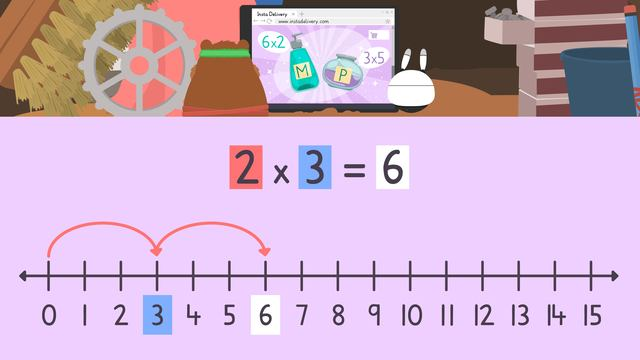# Multiplication on a Number LineRating

Ø 5.0 / 2 ratings

The authorsTeam Digital
Multiplication on a Number Line
CCSS.MATH.CONTENT.3.OA.C.7

## Basics on the topicMultiplication on a Number Line

### In This Video on Number Line Multiplication

Mr. Squeaks and Imani are online shopping, but want to make sure they’re not ordering too many items. They want to use multiplication on a number line to calculate how many items they will be buying. In the end, we see them using their products, and they look dashing! At the end, there is a number line multiplication worksheet for you to practice!

### Multiplication - Number Line Example

You might be wondering, ‘how to use a number line for multiplication?’ Multiplication using number line requires you to follow some steps to set up the problem, and solve it. To do multiplication using a number line:

• Set up a number line for multiplication• Write the multiplication read at the top. Here, we have two times three• The first factor, two, tells you how many times to jump• The second factor, three, tells us how many we are jumping by each time.• Now complete the jumps! For two times three, we are doing two jumps of three, which lands us on our product, sixThis is how you can use a number line model for multiplication.

### Multiplication with a Number Line - Summary

Remember, to do multiplication on number line:

• Set up a number line
• The first factor tells you how many times to jump
• The second factor tells you how many to jump by each time
• The number you land on is the productYou can find a multiplication on a number line worksheet at the end of this.

### TranscriptMultiplication on a Number Line

Mr. Squeaks and Imani are doing some online shopping with instant delivery. Items come in boxes of multiples, and Mr. Squeaks wants to calculate the total before ordering. Let's help them by using multiplication on a number line. When multiplying on a number line, first, identify the factor pair. Here, we have two and three. The first factor, two, tells us how many times we will jump, and the second factor, three, tells us how many we are jumping by each time. Starting from zero, we will do two jumps of three each time. From zero, one jump lands us on three, and two jumps lands us on six. The final number landed on is the product. Two multiplied by three gives a product of six. After taking some time to browse, it looks like Mr. Squeaks is going to order six boxes with two bottles of fur mousse in each box. With a number line ready, what is the factor pair we are multiplying here? It is six and two, meaning we will do six jumps of two each time. If we do six jumps of two, what number do we land on? One jump lands on two. Two jumps land on four. Three jumps land on six. Four jumps land on eight. Five jumps land on ten, and six jumps land on twelve. What is the product of six and two? We landed on twelve, so this is our product. Six times two gives a product of twelve, meaning they will order twelve bottles of fur mousse for Mr. Squeaks. Now they have added Mr. Squeaks fur mousse to their shopping cart, it's time to order Imani some rust free polish! Mr. Squeaks decides to order Imani three boxes with five containers of polish in each. With a number line ready, what is the factor pair we are multiplying? The factor pair is three and five. What is the next step? The next step is to do three jumps of five each. If we do three jumps of five, what number do we land on? When we do three jumps of five, we land on fifteen. What is the product of three and five? The product of three and five is fifteen, meaning they will order fifteen containers of rust free polish for Imani. While Mr. Squeaks and Imani submit their order and wait for instant delivery, let's review! Remember, first identify the factor pair. The first factor tells you the number of jumps and the second factor tells you how many you are jumping by each time. The final number landed on after all jumps are completed is the product. "Wow, they really meant instant delivery, but what is that look, Mr. Squeaks?" "This, Imani, is called fashion!"